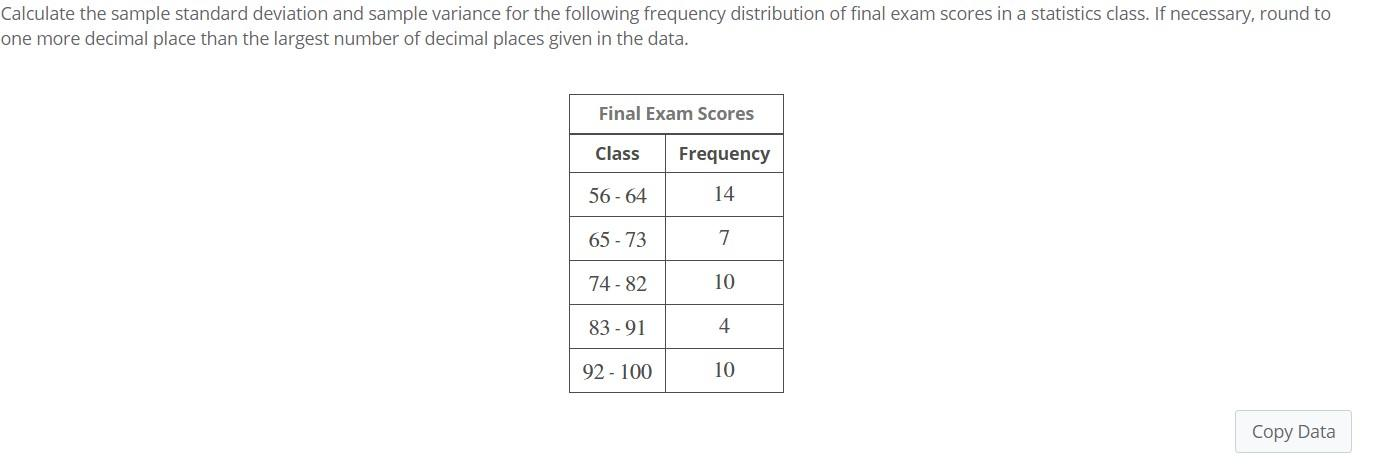# Question Solved1 AnswerCalculate the sample standard deviation and sample variance for the following frequency distribution of final exam scores in a statistics class. If necessary, round to one more decimal place than the largest number of decimal places given in the data. Final Exam Scores Class Frequency 56 - 64 14 65-73 74 - 82 10 83-91 4 92-100 10 Copy DataFOIS4L The Asker · Probability and StatisticsTranscribed Image Text: Calculate the sample standard deviation and sample variance for the following frequency distribution of final exam scores in a statistics class. If necessary, round to one more decimal place than the largest number of decimal places given in the data. Final Exam Scores Class Frequency 56 - 64 14 65-73 74 - 82 10 83-91 4 92-100 10 Copy Data
More
Transcribed Image Text: Calculate the sample standard deviation and sample variance for the following frequency distribution of final exam scores in a statistics class. If necessary, round to one more decimal place than the largest number of decimal places given in the data. Final Exam Scores Class Frequency 56 - 64 14 65-73 74 - 82 10 83-91 4 92-100 10 Copy Data